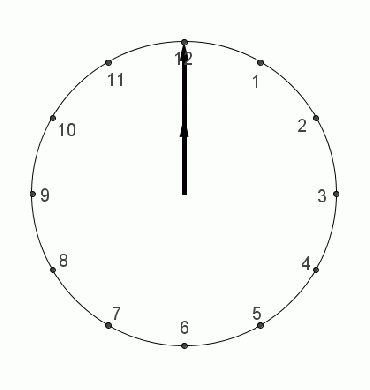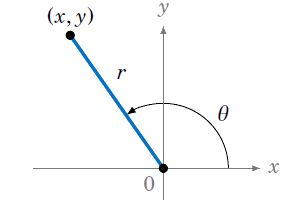Right triangle trigonometry

In elementary geometry you learn a handful of definitions for the different types of angles that you study. Acute angles are between 0° and 90°, right angles are equal to 90°, obtuse angles are between 90° and 180°, and straight angles are equal to 180°.Fig 1. Different types of angles

We also have definitions for acute triangles that add to 90° (complementary), angles between 0° and 180° that add to 180° (supplementary) and angles between 0° and 360° that add to 360° (conjugate).Fig 2. A right triangle

When you have a right triangle, the side opposite to its right angle is called the hypotenuse, and the other two sides are its legs. The Pythagorean Theorem allows you to find the length of the hypotenuse, and with some algebraic manipulation, to calculate the length of any right-angle triangle’s sides given the other two with

$$a^2 + b^2 = c^2$$

If you have a right triangle $\triangle ACB$, you can compute trigonometric functions for its acute angles. If we consider any of the two acute angles, we can find ratios between the legs (or sides) that are adjacent and opposite to the angle. There are six of these ratios, three of them are important to know (sine, cosine, tangent), the remaining others (cosecant, secant, cotangent) are just their inverse.

It’s easy to get tripped up about what these functions do; technically, they’re just ratios between sides of a triangle. The reason why they exist in this form is that they help us formalize the intuition that similar triangles have the same shape but different sizes. Trigonometric functions can be used (among other things) to see by how much similar triangles diverge in size because, really, their corresponding angles are equal (as are the ratios—the trigonometric function(s)—of their corresponding sides).

In practice, at this stage, they’re used to derive the sides of a right triangle through algebraic manipulations that rest on solving for whatever side you want to find, given an initial angle.

When we want to define trigonometric functions for any angle regardless of degree, we can look at them as the hands of a clock spinning around a vertex. Depending on the direction of the rotation, an angle is either positive (moving counter-clockwise) or negative (moving clockwise).Fig 2. Generalization of angles

This opens up the possibility to define trigonometric functions of any angle in terms of the Cartesian coordinates, which is where trigonometry starts to bleed into Calculus and Linear Algebra.Fig 3. Angles in coordinate plane